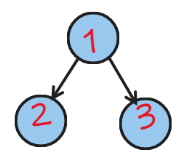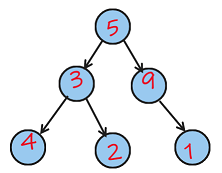New update is available. Click here to update.
Topics

# Left Right Print

Moderate0/80
Average time to solve is 30m
0 upvote## Problem statement

You are given the root of a binary tree. Print the values of the tree in the following way:

i. Print the values of nodes of the last level in this way: print the leftmost value then the rightmost value and continue until all the values of nodes of the last level are printed.

ii. Do the same for the second last level then the third last level until the first level.

There are total ‘N’ nodes, nodes are numbered from 0 to ‘N’-1. The values of nodes are given by an array ‘NUMS’, Where ‘NUMS[ i ]’ = the value of the ‘i’th node. You are also given the level order traversal of the tree in an array ‘SEQUENCE’. ‘SEQUENCE[ i ]’ = -1 denotes a null node.

Example:
``````Input: ‘N’ = 3,  ‘NUMS’ = [1, 2, 3], ‘SEQUENCE’ = [0, 1, 2, -1, -1, -1, -1].
````````````Output: [2, 3, 1]
Printing the last level as the leftmost value then the rightmost value we get = 2, 3
Printing the 2nd last level we get = 1
Hence, the final array is: [2, 3, 1].
``````
Detailed explanation ( Input/output format, Notes, Images )
Constraints :
``````1 <= T <= 10
1 <= N <= 10^5
Sum of ‘N’ <= 10^5
1 <= NUMS[i] <= 10^9

Time Limit: 1 sec
``````
Sample Input 1 :
``````1
6
5 3 9 4 2 1
0 1 2 3 4 -1 5 -1 -1 -1 -1 -1 -1
``````
Sample Output 1 :
``````4 1 2 3 9 5
``````
Explanation Of Sample Input 1 :
``````For the first case:
The tree generated from the edges is:
````````````Starting from the last level printing the leftmost value then the rightmost value we get 4 1 2 3 9 5.
So the final output is [4, 1, 2, 3, 9, 5].
``````
Sample Input 2 :
``````2
3
1 2 3
0 1 2 -1 -1 -1 -1
2
5 11
0 -1 1 -1 -1
``````
Sample Output 2 :
``````2 3 1
11 5
``````Console